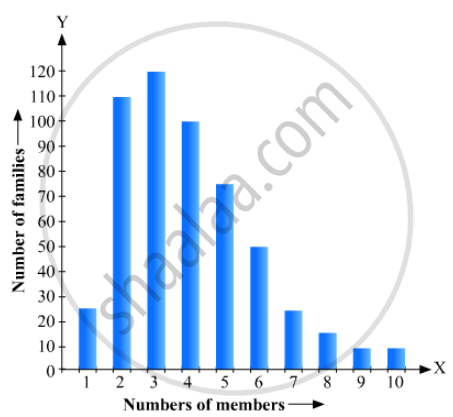# Read the Bar Graph Given in Fig. 23.21 and Answer the Following Questions: (I) What is the Information Given by the Bar Graph? (Ii) What is the Number of Families Having 6 Members? - Mathematics

Read the bar graph given in Fig. 23.21 and answer the following questions:
(i) What is the information given by the bar graph?
(ii) What is the number of families having 6 members?
(iii) How many members per family are there in the maximum number of families? Also tell the number of such families.
(iv) What are the number of members per family for which the number of families are equal? Also, tell the number of such families?#### Solution

(i) It gives the information about the number of families with different number of members in a locality.
(ii) The number of families having 6 members = 50
(iii) 3 members per family are there in the maximum number of families The number of families which have 3 members = 120.
(iv) 9 and 10 are the number of members per family for which the number of families are equal The number of such families is 5.

Concept: Graphical Representation of Data
Is there an error in this question or solution?

#### APPEARS IN

RD Sharma Mathematics for Class 9
Chapter 23 Graphical Representation of Statistical Data
Exercise 23.1 | Q 17 | Page 16

Share